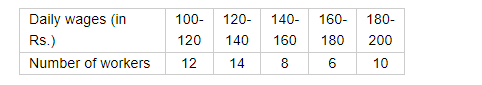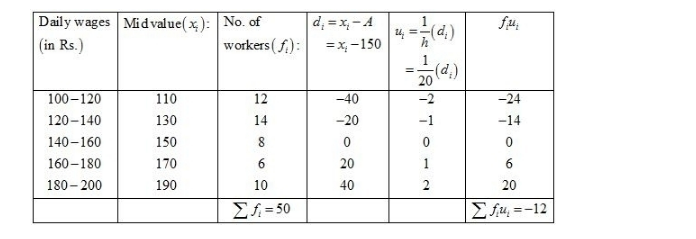# Consider the following distribution of daily wages of 50 workers of a factory.

Question:

Consider the following distribution of daily wages of 50 workers of a factory.Find the mean daily wages of the workers of the factory by using an appropriate method.

Solution:

Let the assumed mean be A = 120 and h = 20.We know that mean, $\bar{X}=A+h\left(\frac{1}{N} \sum f_{i} u_{i}\right)$

Now, we have $N=\sum f_{i}=50, \sum f_{i} u_{i}=-12, h=20$ and $A=150$.

Putting the values in the above formula, we have

$\bar{X}=A+h\left(\frac{1}{N} \sum f_{i} u_{i}\right)$

$=150+20\left(\frac{1}{50} \times(-12)\right)$

$=150-\frac{240}{50}$

$=150-4.8$

$=145.2$

Hence, the mean daily wage of the workers is Rs 145.20.